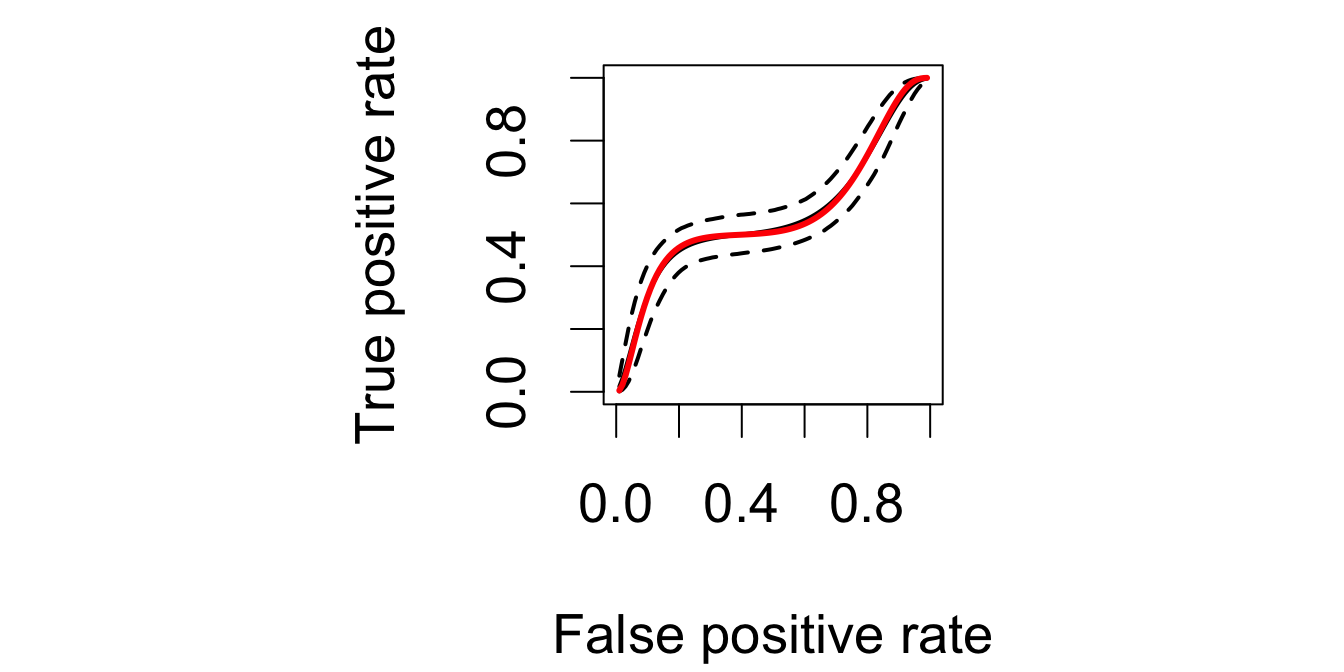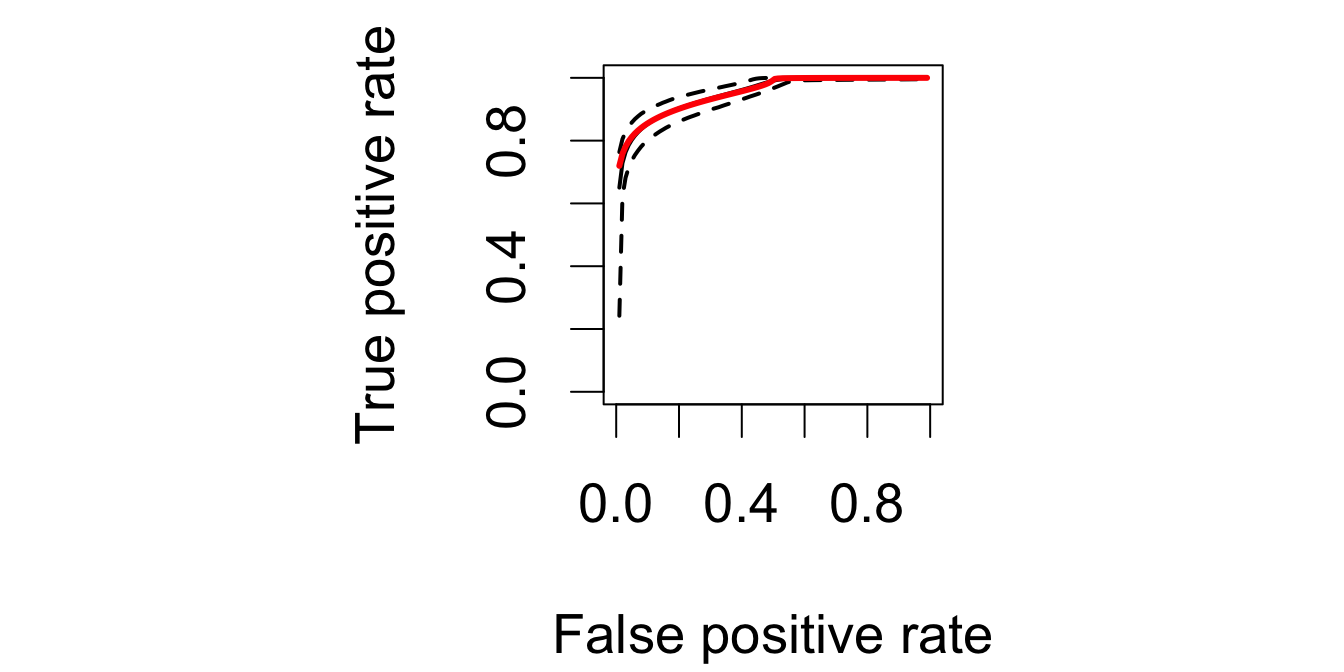Below, I discuss some R code for illustrating how to implement the estimator for the covariate-adjusted ROC curve proposed by Inácio de Carvalho et al. (2013), in a simulated data example; for the interpretation of the quantities computed in this script, the reader is referred to the article. Before running the code chunks below, start by cleaning workspace and install the following packages (if not installed).

rm(list = ls())
if (!require("DPpackage")) install.packages("DPpackage")

For reproducibility reasons, we fix setseed and list below the information about R, the OS, and loaded packages:

set.seed(1)
sessionInfo()
## R version 3.3.2 (2016-10-31)
## Platform: x86_64-apple-darwin13.4.0 (64-bit)
## Running under: macOS Sierra 10.12.1
##
## locale:
##  en_US.UTF-8/en_US.UTF-8/en_US.UTF-8/C/en_US.UTF-8/en_US.UTF-8
##
## attached base packages:
##  splines   stats     graphics  grDevices utils     datasets  methods
##  base
##
## other attached packages:
##  DPpackage_1.1-6 survival_2.39-5 nlme_3.1-128    MASS_7.3-45
##
## loaded via a namespace (and not attached):
##   Rcpp_0.12.5     lattice_0.20-34 digest_0.6.9    assertthat_0.1
##   grid_3.3.2      magrittr_1.5    evaluate_0.10   stringi_1.1.1
##   Matrix_1.2-7.1  rmarkdown_1.1   tools_3.3.2     stringr_1.0.0
##  yaml_2.1.13     htmltools_0.3.5 knitr_1.15      tibble_1.2

In the code chunks below, I follow the 80 characters per line standard. The main routine to be used below is LDDProc which has been coded by Alejandro Jara (ajara@mat.puc.cl) along with the example. We consider one binary predictor and set $$n_D = n_{\bar{D}} = 250$$.

Here are a few auxiliary functions

findq <- function(true.cdf, target, low, upp, epsilon = 0.0000001) {
plow <- true.cdf(low)
pupp <- true.cdf(upp)
pcenter <- true.cdf((upp + low)/2)
err <- abs(pcenter - target)
i <- 0
while(err > epsilon) {
i <- i + 1
if(target < pcenter) {
upp <- (upp + low)/2
pupp <- pcenter
pcenter <- true.cdf((upp + low)/2)
err <- abs(pcenter - target)
}
if(target >= pcenter) {
low <- (upp + low)/2
plow <- pcenter
pcenter <- true.cdf((upp + low)/2)
err <- abs(pcenter - target)
}
}
return((upp+low)/2)
}

true.cdf.nond1 <- function(x)
pnorm(x, 2.1, sqrt(0.0324))

true.cdf.nond2 <- function(x)
0.5 * pnorm(x, 1.85, sqrt(0.005)) + 0.5 * pnorm(x, 2.25, sqrt(0.005))

true.cdf.d1 <- function(x)
0.5 * pnorm(x, 1.95, sqrt(0.005)) + 0.5 * pnorm(x, 2.35, sqrt(0.005))

true.cdf.d2 <- function(x)
pnorm(x, 2.5, sqrt(0.0324))

Let’s now simulate the data:

nsim <- 250
qq <- seq(1, nsim) / (nsim + 1)

y.nond1 <- rep(0,nsim)
for(i in 1:nsim) {
aa <- findq(true.cdf.nond1, qq[i], low = -6, upp = 6, epsilon = 0.0000001)
y.nond1[i] <- aa
}

y.nond2 <- rep(0,nsim)
for(i in 1:nsim) {
aa <- findq(true.cdf.nond2, qq[i], low = -6, upp = 6, epsilon = 0.0000001)
y.nond2[i] <- aa
}

y.nond <- c(y.nond1, y.nond2)
trt.nond <- c(rep(0, nsim), rep(1, nsim))

y.d1 <- rep(0,nsim)
for(i in 1:nsim) {
aa <- findq(true.cdf.d1, qq[i], low = -6, upp = 6, epsilon = 0.0000001)
y.d1[i] <- aa
}

y.d2 <- rep(0,nsim)
for(i in 1:nsim) {
aa <- findq(true.cdf.d2, qq[i], low=-6, upp = 6, epsilon = 0.0000001)
y.d2[i] <- aa
}

y.d <- c(y.d1, y.d2)
trt.d <- c(rep(0, nsim), rep(1, nsim))

Here are the design matrices

z.d <- cbind(rep(1,2*nsim), trt.d)
colnames(z.d) <- c("(Intercept)", "trt")
z.nond <- cbind(rep(1,2*nsim), trt.nond)
colnames(z.nond) <- c("(Intercept)", "trt")

and the design matrix for posterior predictive inference

zpred <- rbind(c(1, 0),c(1, 1))  

In terms of prior information and MCMC parameters, we set

# Prior information
prior <- list(a0 = 10, b0 = 1, nu = 4, m0 = rep(0, 2), S0 = diag(100, 2),
psiinv = diag(1, 2), tau1 = 6.01, taus1 = 6.01, taus2 = 2.01)

# Initial state
state <- NULL

# MCMC parameters
nburn <- 5000
nsave <- 5000
nskip <- 4
ndisplay <- 500
mcmc <- list(nburn = nburn, nsave = nsave, nskip = nskip, ndisplay = ndisplay)

We are thus ready to fit the model

fit <- LDDProc(y.d = y.d, z.d = z.d, y.nond = y.nond, z.nond = z.nond,
zpred.d = zpred, prior.d = prior, prior.nond = prior,
mcmc = mcmc, state = state, status = TRUE,
compute.band = TRUE)
##
## Fitting the model for the non-diseased population
##
## MCMC scan 500 of 5000 (CPU time: 64.285 s)
## MCMC scan 1000 of 5000 (CPU time: 134.257 s)
## MCMC scan 1500 of 5000 (CPU time: 202.853 s)
## MCMC scan 2000 of 5000 (CPU time: 266.044 s)
## MCMC scan 2500 of 5000 (CPU time: 335.537 s)
## MCMC scan 3000 of 5000 (CPU time: 406.351 s)
## MCMC scan 3500 of 5000 (CPU time: 479.545 s)
## MCMC scan 4000 of 5000 (CPU time: 550.807 s)
## MCMC scan 4500 of 5000 (CPU time: 620.629 s)
## MCMC scan 5000 of 5000 (CPU time: 687.532 s)
##
##
## Fitting the model for the diseased population
##
## MCMC scan 500 of 5000 (CPU time: 91.921 s)
## MCMC scan 1000 of 5000 (CPU time: 178.037 s)
## MCMC scan 1500 of 5000 (CPU time: 266.393 s)
## MCMC scan 2000 of 5000 (CPU time: 350.146 s)
## MCMC scan 2500 of 5000 (CPU time: 434.584 s)
## MCMC scan 3000 of 5000 (CPU time: 518.345 s)
## MCMC scan 3500 of 5000 (CPU time: 601.617 s)
## MCMC scan 4000 of 5000 (CPU time: 667.235 s)
## MCMC scan 4500 of 5000 (CPU time: 735.398 s)
## MCMC scan 5000 of 5000 (CPU time: 800.845 s)

To plot the covariate-adjusted ROC curve at x = c(1, 0), along with the true curve, use the following code:

par(cex = 1.7, mar = c(4.1, 4.1, 1, 1), pty = 's')

plot(fit$rocgrid, fit$rocp.h[1, ], type = "l", lty = 2, lwd = 2,
xlim = c(0, 1), ylim = c(0, 1),
xlab = "False positive rate", ylab = "True positive rate")
lines(fit$rocgrid, fit$rocp.l[1, ], lty = 2, lwd = 2)
lines(fit$rocgrid, fit$rocp.m[1, ], lty = 1, lwd = 2)

nn <- length(fit$rocgrid) tt <- rep(0, nn) for(i in 1:nn) tt[i] <- findq(true.cdf.nond1, 1 - fit$rocgrid[i], low = -6, upp = 6,
epsilon = 0.0000001)

true.roc1 <- 1.0 - true.cdf.d1(tt)
lines(fit$rocgrid, true.roc1, lty = 1, lwd = 3, col = "red")Fig. 1: Covariate-adjusted ROC curve at $$\mathbf{x} = (1, 0)'$$ along with true curve (red line) And to plot the covariate-adjusted ROC curve for x = c(1, 1), along with the true curve, use the code par(cex = 1.7, mar = c(4.1, 4.1, 1, 1), pty = 's') plot(fit$rocgrid, fit$rocp.h[2, ], type = "l", lty = 2, lwd = 2, xlim = c(0, 1), ylim = c(0, 1), xlab = "False positive rate", ylab = "True positive rate") lines(fit$rocgrid,fit$rocp.l[2, ], lty = 2, lwd = 2) lines(fit$rocgrid,fit$rocp.m[2, ], lty = 1, lwd = 2) nn <- length(fit$rocgrid)
tt <- rep(0, nn)
for(i in 1:nn)
tt[i] <- findq(true.cdf.nond2, 1-fit$rocgrid[i], low = -6, upp = 6, epsilon = 0.0000001) true.roc2 <- 1.0 - true.cdf.d2(tt) lines(fit$rocgrid,true.roc2, lty = 1, lwd = 3, col = "red")Fig. 2: Covariate-adjusted ROC curve at $$\mathbf{x} = (1, 1)'$$ along with true curve (red line)